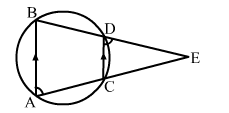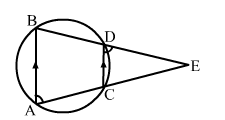# In the given figure, AB and CD are two parallel chords of a circle. If BDE and ACE are straight lines, intersecting at E,

Question:

In the given figure, AB and CD are two parallel chords of a circle. If BDE and ACE are straight lines, intersecting at E, prove that AEB is isosceles.Solution:AB and CD are two parallel chords of a circle. BDE and ACE are two straight lines that intersect at E.
If one side of a cyclic quadrilateral is produced, then the exterior angle is equal to the interior opposite angle.
∴ Exterior ∠EDC = ∠A   ...(i)
Exterior ∠DCE = ∠B    ...(ii)
Also, AB parallel to CD.
Then, ∠EDC = ∠B  (Corresponding angles)
and ∠DCE = ∠A  (Corresponding angles)
∴ ∠A = ∠B     [From(i) amd (ii)]
Hence, ΔAEB is isosceles.